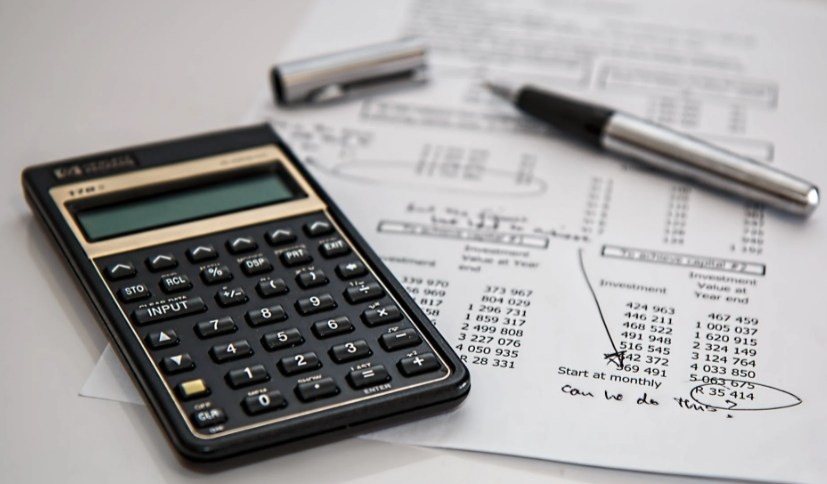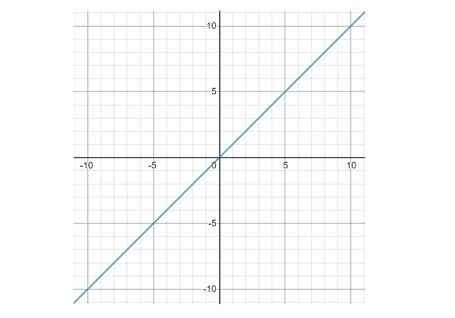# How to Convert Different Types of Numbers in Standard Form

In mathematics, the standard form is used to easily read and write numbers that are very large or small in all fields of science. Let's learn easy math tricks to convert numbers,  linear equations and polynomials to standard form with lots of examples.Standard form conversion calculator

## How to Convert Different Types of Numbers to Standard Form?

Mathematics is used to study measurements, numbers, and space. It plays a vital role in all aspects of our life.

In mathematics, the standard form is used widely to reduce the difficulty of reading and writing a very large number or a very small number, like it is very difficult to read a number like 345678900000000 or 0.0000000000000030202. So, we use larger or smaller numbers in standard form.

Standard form is used in many other branches of science like physics, statistics, chemistry, etc. It is very helpful in reading and writing numbers which are very large or small in all fields of science.

In this post, we will learn various methods to convert in standard form along with a lot of examples.

## What is Standard Form?

Standard form is that form in which any number from 1 to 9 can be written in decimal form multiplied by the power of 10.

For example, 345678900 can be written in standard form as 3.46 x 108 and 0.0000030202 can be written in standard form as 3.02 x 10-6. For the perfect result of your problem related to standard form use a standard form calculator.

## Methods to convert numbers, linear equation, and polynomial in Standard Form

There are various methods to convert numbers or equations in standard form.

### Conversion of Numbers

There are two ways to convert a number into a standard form. The criteria of converting a large number in standard form are totally different for the conversion of small numbers.

For larger numbers

For the conversion of the larger numbers into standard form, we put a decimal point after the first significant digit and count all the digits after point from left to right and write the results in the power of 10. The power number will be positive.

Example

Convert 345678900 in standard form.

Solution

Step 1: Place the decimal point after the first significant digit.

3.45678900

Step 2: Count the digits after point form left to right and write the results in the power of 10.

3.45678900 x 108

Step 3: Round the number up to three decimal points.

3.457 x 108

For smaller numbers

For the conversion of the smaller numbers into standard form, we put a decimal point after the first significant digit and count all the digits before the point from right to left and write the results in the power of 10. The power number will be negative.

Example

Convert 0.0000030202 in standard form.

Solution

Step 1: Place the decimal point after the first significant digit.

0000003.0202

Step 2: Count the digits before point form right to left and write the results in the power of 10.

0000003.02 x 10-6

Step 3: Ignore the leading zeros.

3.02 x 10-6

An online standard notation calculator can also be used for the accurate results of the given larger or smaller numbers in the notation of the standard form.

### Conversion of linear equationsFor the conversion of a linear equation into the standard form we must have an equation that is linear means all the powers of the variables should be one and in form of Ax + By = C.

In the above linear equation, x and y are variables, and A, B, and C are the coefficient of the linear equation. To convert any linear equation in the standard form you must follow some instructions such as,

All the variables should be on the same side of the equation, all the coefficients should be integers and if the coefficients are in fraction or decimals convert them into integers, the first variable of the equation must be positive such as x, and all the coefficients have only 1 and itself as a common factor.

Example

Convert the given linear equation in Standard form 12/3 y = 8x + 16.

Solution

Step 1: Place the variables on the same side of the equation.

-8x + 12/3 y = 16

Step 2: Convert the fraction into an integer.

-8x + 4y = 16

Step 3: The first variable must be positive, multiply by -1 on both sides of the equation.

-1(-8x + 4y) = 16(-1)

8x - 4y = -16

Step 4: Coefficients must have no common factors other than one, divide by 4 on both sides.

8/4 x – 4/4 y = -16/4

2x – y = -4

Hence, the given linear equation is converted in standard form.

### Conversion of Polynomials

For the conversion of the polynomial equation into the standard form, we must have an equation that is not linear means all the powers of the variables should be greater than one and in the form of

Ax3 + Bx2 + Cx = D.

In the above linear equation x3, x2, and x are variables, and A, B, C, and D are the coefficient of the polynomial equation. To convert any polynomial equation in the standard form you must follow some instructions such as,

All the variables or powers of x should be on the same side of the equation, all the coefficients should be integers and if the coefficients are in fraction or decimals convert them into integers, the first variable or power of the equation must be positive such as x3, and all the coefficients have only 1 and itself as a common factor.

Example

Convert the given linear equation in Standard form -4x3 - 16/2 x4 = - 8x + 16.

Solution

Step 1: Place the variables or powers of x on the same side of the equation.

-4x3 - 16/2 x4 + 8x = 16

Step 2: All the coefficients must be integers, convert the fraction into an integer.

-4x3 - 8x4 + 8x = 16

Step 3: Arrange the equation from a higher power to lower power.

-8x4 - 4x3 + 8x = 16

Step 4: The first variable must be positive, multiply by -1 on both sides of the equation.

-(-8x4 - 4x3 + 8x) = -16

8x4 + 4x3 - 8x = -16

Step 5: Coefficients must have no common factors other than one, divide by 4 on both sides.

8/4x4 + 4/4x3 – 8/4x = -16/4

2x4 + x3 - 2x = -4

Hence, the given Polynomial equation is converted in standard form.

## Summary

In this post, we have gone through all the basics required to convert a number, linear equation, or polynomial in standard form. Standard form is used for those larger or smaller numbers that are in a larger order.